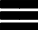Krivalar TutorialsKrivalar Tutorials

# Java Program to Find Factorial of a Number

Factorial of a number is the multiplication of a number with all the integers less than it. Factorial of a number is written as the number followed by exclamation symbol !. For Example, Factorial of 4 is written as 4!.

```n! = n * (n - 1)!
0! = 1
1! = 1
4! = 4 * 3 * 2 * 1 = 24
5! = 5 * 4 * 3 * 2 * 1 = 120
6! = 6 * 5 * 4 * 3 * 2 * 1 = 720
```

## Algorithm

1. Set factorial as 1
2. Store the Number for which you want to find the factorial in a variable say i
3. Multiply factorial * i and store in factorial
4. Decrement i and store in i itself
5. Repeat the above steps until i=1

## Factorial of a Number - Java Program

``````public class FactorialOfANumber {
public static void main (String args[]) {

int num = 5;
System.out.print("Factorial of the Number " + num + " = ");
int factorial=1;

for(int i = num; i >= 1 ; i--) {
System.out.print(""+ i );

factorial = factorial * i;

if(i!=1) System.out.print(" * ");
}
System.out.print("=" + factorial);
}
}
``````

Output of the Java program would be:

```
Factorial of the Number 5 = 5 * 4 * 3 * 2 * 1=120

```

## Factorial of Some Numbers

NumberCalculationValue
0 factorial11
1 factorial11
2 factorial2 * 12
3 factorial3 * 2 * 16
4 factorial4 * 3!24
5 factorial5 * 4!120
6 factorial6 * 5!720
7 factorial7 * 6!5,040
8 factorial8 * 7!40,320
9 factorial9 * 8!362,880
10 factorial10 * 9!3,628,800
11 factorial11 * 10!3,991,680
100 factorial100 * 99!9.332622e+157